PHY_10_64_V1_P_Faraday law of induction
Оценка 5

# PHY_10_64_V1_P_Faraday law of induction

Оценка 5
ppt
08.05.2020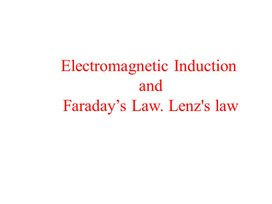PHY_10_64_V1_P_Faraday law of induction.ppt

Electromagnetic Induction and Faraday’s Law. Lenz's law

## 10.4.2.1 – to explain the occurrence of electromotive force when changing the magnetic flux; 10.4.2.2 – to explain the Lentz's law; Lesson Objectives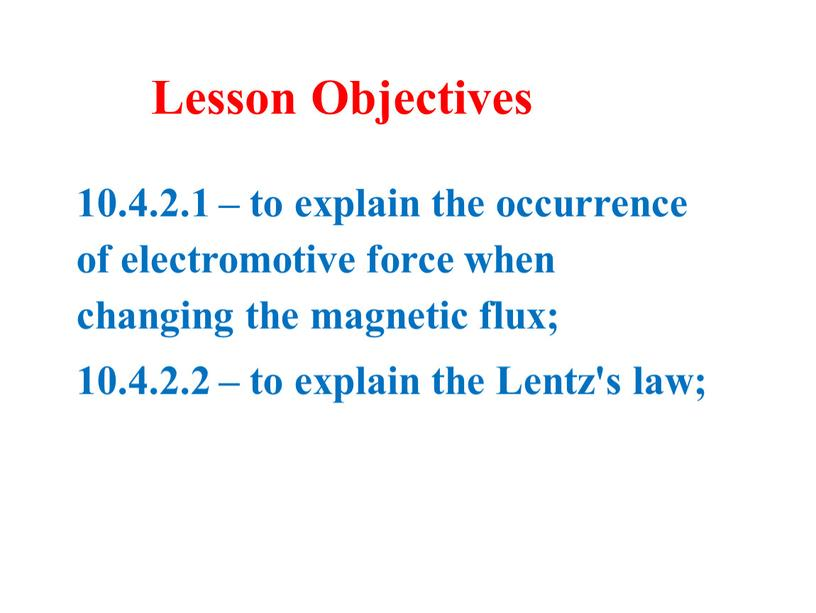10.4.2.1 – to explain the occurrence of electromotive force when changing the magnetic flux;
10.4.2.2 – to explain the Lentz's law;

Lesson Objectives

Course of the lesson

Electromagnetism
Faraday’s Law
Lenz’ Law
Applications

## Spring 2008 5 Induced Current The next part of the story is that a changing magnetic field produces an electric current in a loop surrounding…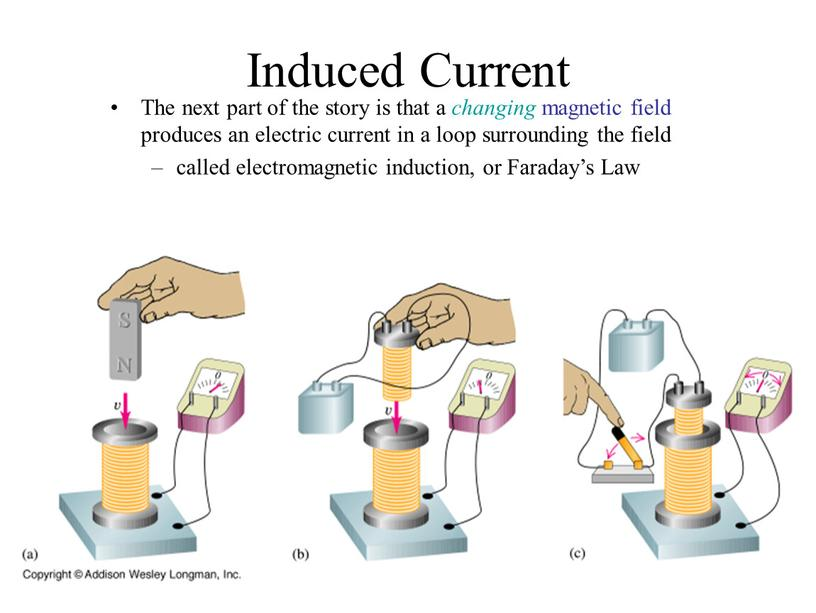Spring 2008

5

Induced Current

The next part of the story is that a changing magnetic field produces an electric current in a loop surrounding the field
called electromagnetic induction, or Faraday’s Law

Faraday’s Law of Induction; Lenz’s Law

The induced emf in a wire loop is proportional to the rate of change of magnetic flux through the loop.
Magnetic flux:

(on formula sheet)

Unit of magnetic flux: weber, Wb.
1 Wb = 1 T·m2

## This drawing shows the variables in the flux equation: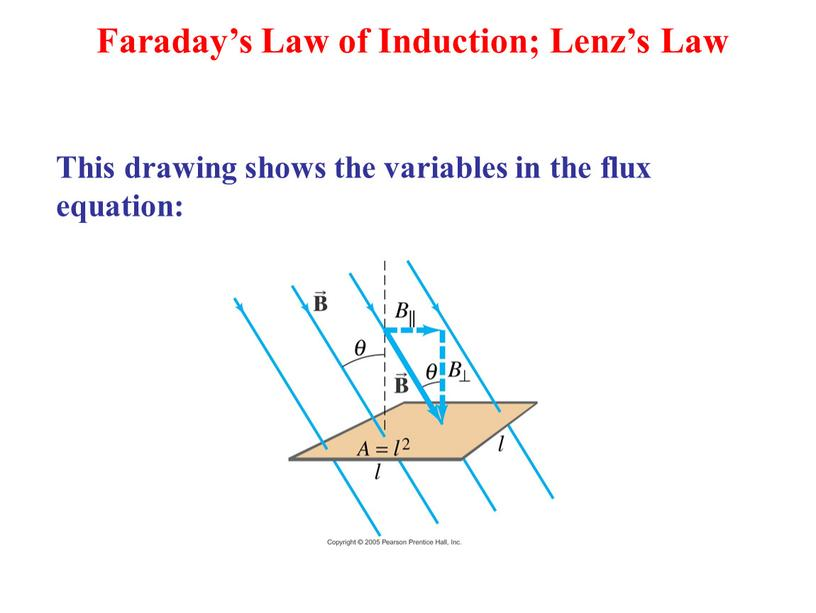This drawing shows the variables in the flux equation:

Faraday’s Law of Induction; Lenz’s Law

## Faraday’s law of induction: [1 loop] (on formula sheet) [N loops]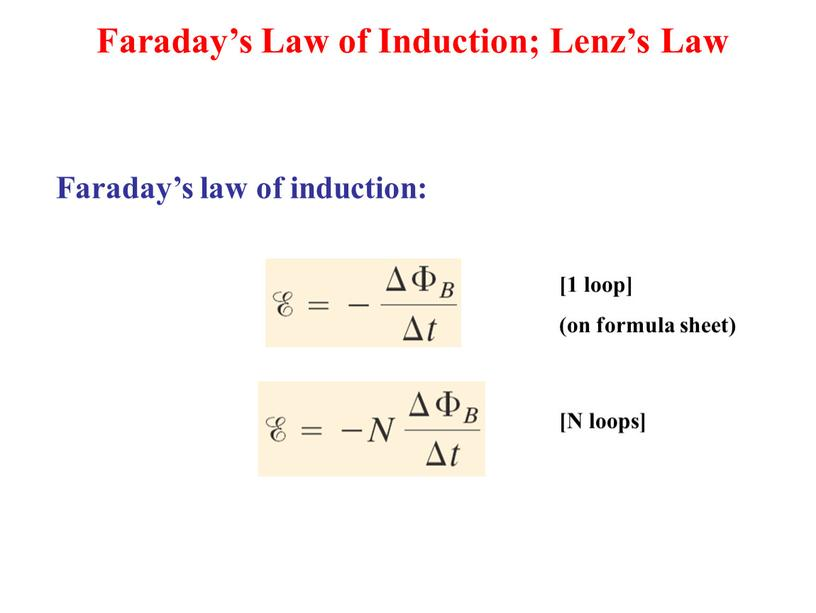Faraday’s law of induction:

[1 loop]
(on formula sheet)

[N loops]

Faraday’s Law of Induction; Lenz’s Law

The minus sign gives the direction of the induced Emf:
An induced Emf always gives rise to a current whose magnetic field opposes the original change in flux (Lenz’s Law).

Faraday’s Law of Induction; Lenz’s Law

In (a) the magnetic field and flux are increasing. The current moves in the direction to oppose that – to decrease the magnetic field.
In (b) the magnetic field and flux are decreasing. Again, the current moves in the direction to oppose that.
In (c) there is no change in flux, so there is no induced emf.

## Induced EMF Therefore, a changing magnetic field induces an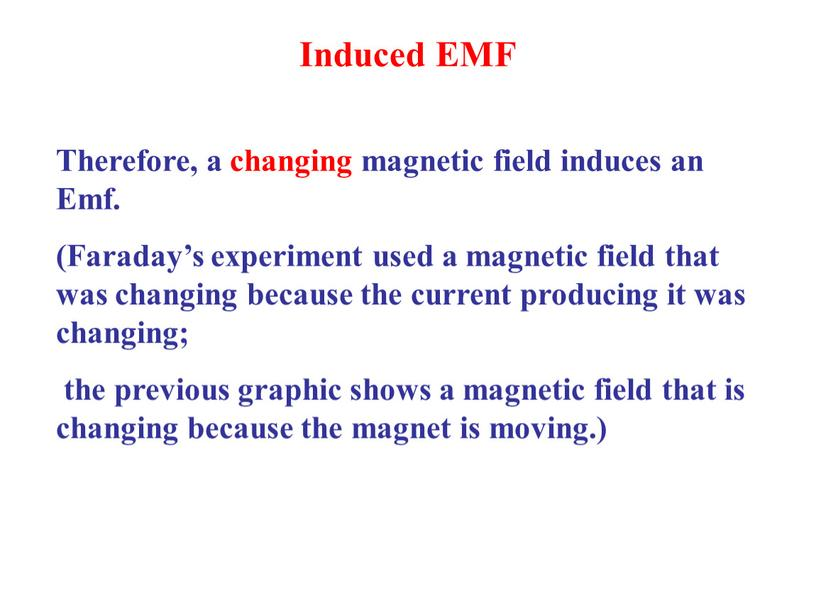Induced EMF

Therefore, a changing magnetic field induces an Emf.
(Faraday’s experiment used a magnetic field that was changing because the current producing it was changing;
the previous graphic shows a magnetic field that is changing because the magnet is moving.)

## Magnetic flux will change if the area of the loop changes: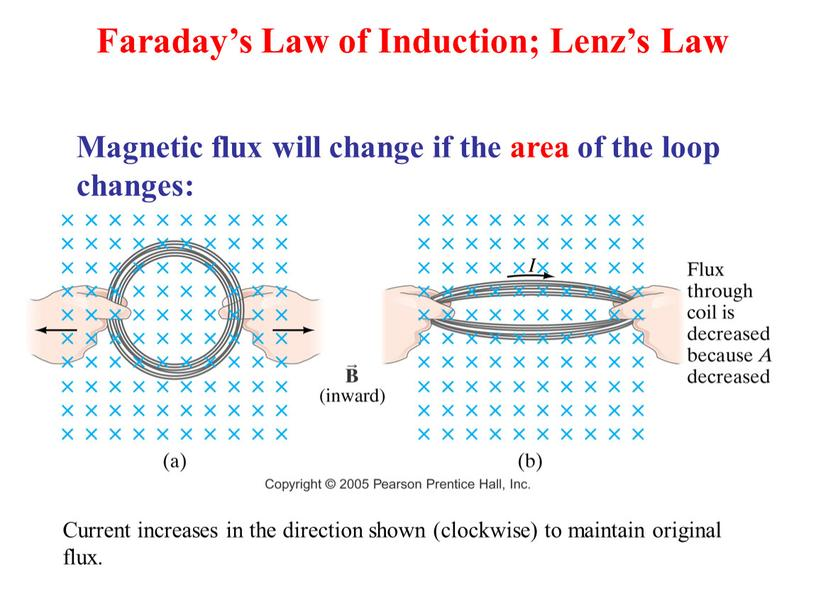Magnetic flux will change if the area of the loop changes:

Faraday’s Law of Induction; Lenz’s Law

Current increases in the direction shown (clockwise) to maintain original flux.

## Magnetic flux will change if the angle between the loop and the field changes: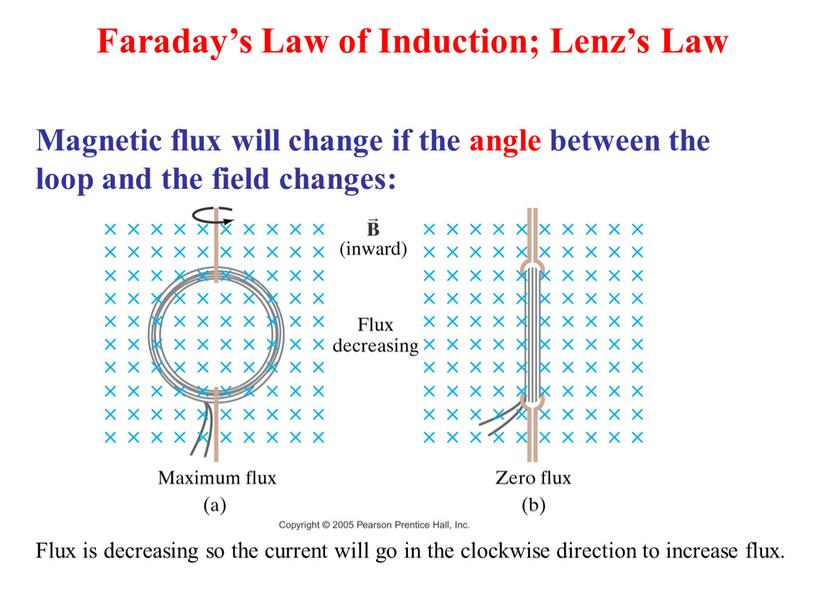Magnetic flux will change if the angle between the loop and the field changes:

Faraday’s Law of Induction; Lenz’s Law

Flux is decreasing so the current will go in the clockwise direction to increase flux.

## Problem Solving: Lenz’s Law Determine whether the magnetic flux is increasing, decreasing, or unchanged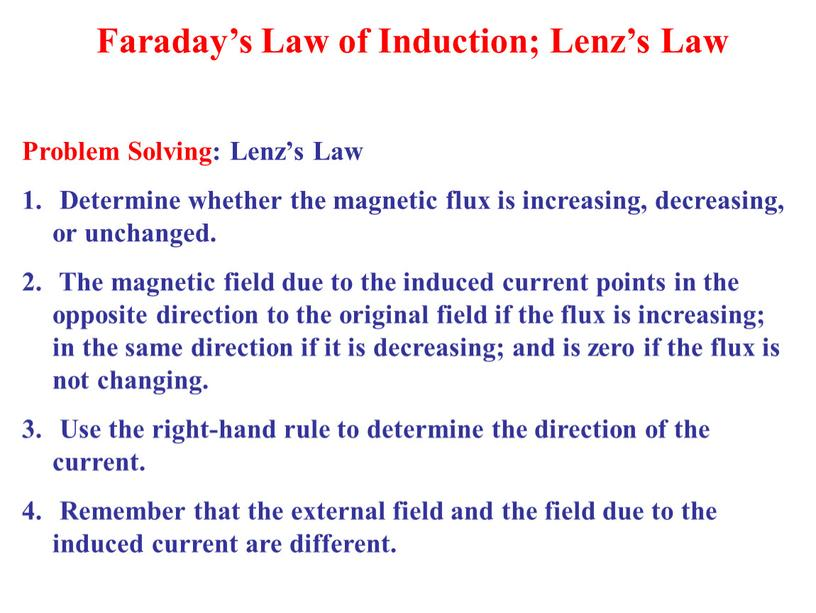Problem Solving: Lenz’s Law
Determine whether the magnetic flux is increasing, decreasing, or unchanged.
The magnetic field due to the induced current points in the opposite direction to the original field if the flux is increasing; in the same direction if it is decreasing; and is zero if the flux is not changing.
Use the right-hand rule to determine the direction of the current.
Remember that the external field and the field due to the induced current are different.

Faraday’s Law of Induction; Lenz’s Law

Скачать файл